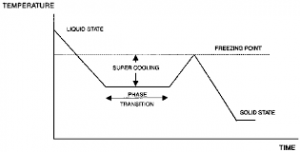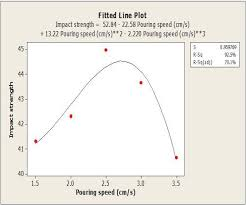## How to Calculate and Solve for Relative Freezing Time | Foundry TechnologyThe image above represents relative freezing time.

To compute for relative freezing time, four essential parameters are needed and these parameters are Constant (L), Constant (C), Volume of Riser/Volume of Casting (y) and Relative Contraction of Freezing (B).

The formula for calculating relative freezing time:

x = L / y – B + C

Where:

x = Relative Freezing Time
y = Volume of Riser / Volume of Casting
B = Relative Contraction on Freezing
C = Constant
L = Constant

Let’s solve an example;
Find the relative freezing time when the volume of riser/volume of casting is 26, the relative contraction on freezing is 21, the constant is 8 and the constant is 11.

This implies that;

y = Volume of Riser / Volume of Casting = 26
B = Relative Contraction on Freezing = 21
C = Constant = 11
L = Constant = 8

x = L / y – B + C
x = 8 / 26 – 21 + 11
x = 8 / 5 + 11
x = 1.6 + 11
x = 12.6

Therefore, the relative freezing time is 12.6 s.

Calculating the Constant (L) when the Relative Freezing Time, Constant (C), the Volume of riser/Volume of Casting and the Relative Contraction on Freezing is Given.

L = x (y – B) – C

Where;

L = Constant
x = Relative Freezing Time
y = Volume of riser / Volume of Casting
B = Relative Contraction on Freezing
C = Constant

Let’s solve an example;
Find the Constant when the relative freezing time is 24, the volume of riser / volume of casting is 14, the relative contraction on freezing is 8 and the constant is 10.

This implies that;

x = Relative Freezing Time = 24
y = Volume of riser / Volume of Casting = 14
B = Relative Contraction on Freezing = 8
C = Constant = 10

L = x (y -B) – C
L = 24 (14 – 8) – 10
L = 24 (6) – 10
L = 144 – 10
L = 134

Therefore, the constant is 134.

## How to Calculate and Solve for Pouring Speed | Design of Gating SystemThe image above represents pouring speed.

To compute for poring speed, three essential parameters are needed and these parameters are Co-efficient for friction (μ), Acceleration due to Gravity (g) and Rated head static (Hs).

The formula for calculating pouring speed:

v = μ√(2gHs)

Where:

v = Pouring Speed
μ = Co-efficient for Friction
g = Acceleration due to Gravity

Let’s solve an example;
Find the pouring speed when the co-efficient for friction is 14, acceleration due to gravity is 9 and rated static head is 16.

This implies that;

μ = Co-efficient for Friction = 14
g = Acceleration due to Gravity = 9
Hs = Rated Static Head = 16

v = μ√(2gHs)
v = 14 x √(2 x 9 x 16)
v = 14 x √(288)
v = 14 x 16.97
v = 237.58

Therefore, the pouring speed is 237.58 m/s.

Calculating the Co-efficient for Friction when the Pouring Speed, the Acceleration due to Gravity and the Rated Static Head is Given.

μ = v / √(2gHs)

Where;

μ = Co-efficient for Friction
v = Pouring Speed
g = Acceleration due to Gravity

Let’s solve an example;
Find the co-efficient for friction when the pouring speed is 24, the acceleration due to gravity is 9 and the rated static head is 11.

This implies that;

v = Pouring Speed = 24
g = Acceleration due to Gravity = 9
Hs = Rated Static Head = 11

μ = v / √(2gHs)
μ = 24 / √(2 x 9 x 11)
μ = 24 / √198
μ = 24 / 14.07
μ = 1.70

Therefore, the co-efficient for friction is 1.70.